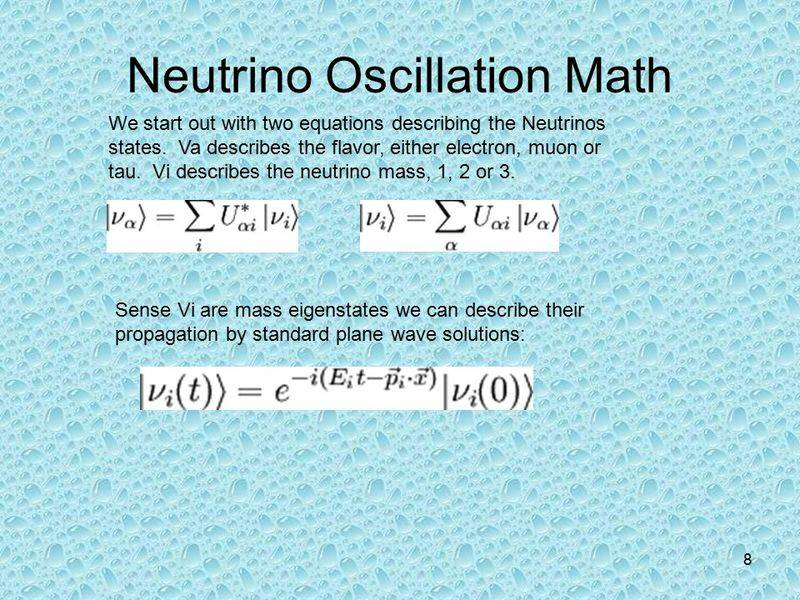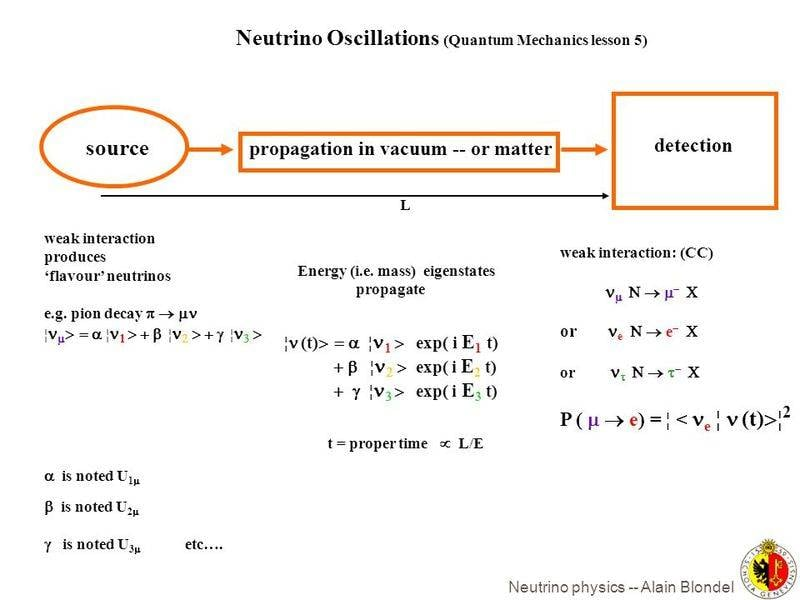# Neutrino mass

• I
Kiley
Why is there an assumption that if neutrinos didn't have mass they would move at the speed of light? and how does the fact they oscillate prove they have mass?

Gold Member
Why is there an assumption that if neutrinos didn't have mass they would move at the speed of light
Because of the $E^2 -p^2 = m^2$
When the mass is 0, then the particle moves at the speed of light and there is no reference frame where it appears at rest.

and how does the fact they oscillate prove they have mass?
Because the oscillation pattern appears with the mass-squared difference between the neutrinos... if they are massless (or even mass-degenerate) there wouldn't be any oscillation visible.

•Spinnor
Staff Emeritus
Homework Helper
Gold Member
2021 Award
It is not just neutrinos. Anything massless in relativity moves at the speed of light, this follows directly from special relativity.

The oscillation phase in neutrino oscillations is directly proportional to the difference between the squares of the masses of the different neutrino mass eigenstates. This means that at most one out of three neutrino masses can be zero.

•fresh_42
Staff Emeritus
Gold Member
Why is there an assumption that if neutrinos didn't have mass they would move at the speed of light? and how does the fact they oscillate prove they have mass?
Any massless particle is required to travel at c in a vacuum. Massless neutrinos would travel at c for the same reason massless photons do.

The oscillations occur because the the wave length of three mass states (electron, muon, and tau) are different, and thus shift in phase with respect to each other. This phase shift is what creates the oscillation. But for the wavelengths to be different, the mass of the three types of neutrinos have to be different, which in turn means they must be finite and non-zero.

Kiley
Thank you all for your replies. Could you recommend any literature of the subject?

Mentor
A suitable introduction to neutrino oscillations depends on how much quantum mechanics you know. Most of the stuff I find with a Google search for "neutrino mass and oscillations" seems to be reviews for physicists who already know something about particle physics and are comfortable with Dirac-bracket notation, superposition of states, etc. Maybe try this on for size, starting with section 4 on page 3:

http://web.mit.edu/shawest/Public/8.06/termPaperDraft.pdf

Kiley
Thank you so much Jtbell, this is very helpful! :)

Staff Emeritus
Homework Helper
Gold Member
2021 Award
three mass states (electron, muon, and tau) are different
Some small bit of nit-picking. The electron muon and tau states are not the neutrino mass states. In fact, it is crucial for oscillations that not only the masses of the mass eigenstates are different, but also that the flavour states, i.e., the electron muon and tau neutrino states, are not equivalent to the mass states but instead are linear combinations of the mass states.

kimmm
Some small bit of nit-picking. The electron muon and tau states are not the neutrino mass states. In fact, it is crucial for oscillations that not only the masses of the mass eigenstates are different, but also that the flavour states, i.e., the electron muon and tau neutrino states, are not equivalent to the mass states but instead are linear combinations of the mass states.
The mass eigenstates are the HamiltonIan eigenstates,but what about the flavour eigenstates,which operator's eigenstates they are?

Gold Member
Some small bit of nit-picking. The electron muon and tau states are not the neutrino mass states. In fact, it is crucial for oscillations that not only the masses of the mass eigenstates are different, but also that the flavour states, i.e., the electron muon and tau neutrino states, are not equivalent to the mass states but instead are linear combinations of the mass states.

The above has confused me for a while, are there any other quantum phenomena in nature that have similar physics to the above?

Thanks!

Staff Emeritus
Homework Helper
Gold Member
2021 Award
The above has confused me for a while, are there any other quantum phenomena in nature that have similar physics to the above?

Thanks!
Quarks. The difference in the quark sector is that the masses are so different that any interference between the mass eigenstates quickly is subject to decoherence. This is why you have W interactions changing the quark generations.

In terms of quantum oscillations, the mathematics is completely analogous to having a spin precessing in a magnetic field that is not parallel to the direction you are measuring the spin component in. For example, you can have a spin-1/2 particle and measure its x-component to be positive at t = 0. Applying a magnetic field in the z-direction, the probability to measure a positive x-component will oscillate between 0 and 1.

•Spinnor
kimmm
Quarks. The difference in the quark sector is that the masses are so different that any interference between the mass eigenstates quickly is subject to decoherence. This is why you have W interactions changing the quark generations.

In terms of quantum oscillations, the mathematics is completely analogous to having a spin precessing in a magnetic field that is not parallel to the direction you are measuring the spin component in. For example, you can have a spin-1/2 particle and measure its x-component to be positive at t = 0. Applying a magnetic field in the z-direction, the probability to measure a positive x-component will oscillate between 0 and 1.
Can I ask a question about the mass eigenstates and flavor eigenstates?
the thing that I understand is that mass eigenstates do not oscillate but the flavors do,and the flavor eigenstates are the superposition of mass eigenstates,and they relate together with a unitary transformation matrix called PMNS,and still we do not know the value of few of neutrino parameters in this matrix, if we find the value of these parametes do we can find the exact value of the neutrinos,like the mass of the electron neutrino or muon neutrinos?
indeed I think I misunderstood the qunatum interpretation of this phenomena,can you help me to understand it?
thanks.

Last edited:
Mentor
like the mass of the electron neutrino or muon neutrinos
There is no such thing.
The electron neutrino is a superposition of the three mass eigenstates. It doesn't have a well-defined mass. It only has an expectation value for the mass.

•Spinnor
kimmm
There is no such thing.
The electron neutrino is a superposition of the three mass eigenstates. It doesn't have a well-defined mass. It only has an expectation value for the mass.
thanks for the reply but still I do not understand the quantum phenomena,so how the superposition is defined by PMNS matrix,and if we know the values of the matrix elements we can not find the definite masses, I mean by mathematics, can you help me to understand how a particle can not have a definite mass?

Last edited:
Staff Emeritus
Homework Helper
Gold Member
2021 Award
and if we know the values of the matrix elements we can not find the definite masses
We can. Those are the masses of the mass eigenstates. General linear combinations of those do not have a definite mass.

It is no different from whatever quantum system. You can have energy eigenstates that have a definite energy and you can have linear combinations of energy eigenstates that, generally, do not.

Gold Member
So I did a Google image search, "the mathematics of neutrino oscillation" and came up with the following image,What more, if anything, than the above is needed to understand neutrino oscillation physics?

Edit, the above just looks like a change of basis?

This is all of it?Thanks!

#### Attachments

Last edited:
kimmm
We can. Those are the masses of the mass eigenstates. General linear combinations of those do not have a definite mass.

So we will never know the exact masses of neutrinos(flavor ones) , as they define by superposition of states?
but just the mass eigenstates by the improvements of experiments,the normal hierarchy or inverted I mean.
is that right?

Staff Emeritus
Homework Helper
Gold Member
2021 Award
So we will never know the exact masses of neutrinos(flavor ones) , as they define by superposition of states?
It is not a question of not knowing the masses of the flavour eigenstates, it is a matter of the flavour eigenstates not having definite masses.

Staff Emeritus
Homework Helper
Gold Member
2021 Award
What more, if anything, than the above is needed to understand neutrino oscillation physics?
That depends on what you put into the word "understand". Effectively, it is just the evolution of a three-state quantum system.

kimmm
It is not a question of not knowing the masses of the flavour eigenstates, it is a matter of the flavour eigenstates not having definite masses.